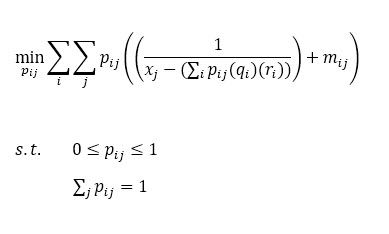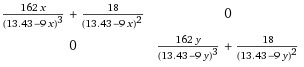# DCP Programming error: {convex} .* {convex}

(Benyamin T) #1

I should minimize a function for my research which is convex at least in its 2-D line plot. My objective function is:

cvx_begin
variable x(n)
minimize (sum (((pow_p(x,3)).*(inv_pos((x./a) - c)))...
+((inv_pos((x./a) - c)))))
subject to
0 <= x <= 1e9
cvx_end


a and c are constants.
I’ve gotten {convex} .* {convex} error for this. I’m somehow familiar with DCP ruleset, but I don’t know how I should make this compatible with DCP rules.

(Mark L. Stone) #2

Looking at it in one dimension, which I think trivially generalizes to n dimensions, the first term boils down to a*x^3/(x-a*c), for x >= max(a*c,0), which presuming a >= 0, is convex on this region, as can be seen from the 2nd derivative… I’ll leave it to someone else to determine whether this can be handled in CVX, for instance with some combination of rotated quadratic (Lorentz) cones.

(Erling D.Andersen) #3

Assuming a is a positive constant then what about :

\begin{array}{rcl} \min & s^2 + \frac{1}{t} & \\ \mbox{st} & s^{2/3} t^{1/3} \geq |x| & \\ & s \geq 0 & \\ & t= x-ac \geq 0 & \\ & 0 \leq x \leq 1e9 \\ \end{array}

generalized to the n dimensional case.

The first constraint is power cone. Not sure if cvx can deal with that but Mosek v9 can.

Note the big upper bound is going to hurt performance and is better avoid if possible.

(Mark L. Stone) #4

CVX does not support “native” power cones. That would be a nice enhancement, but given the paucity of CVX development in recent years, I wouldn’t count on such capability being forthcoming.

So if this can be written in CVX, I believe it will have to be using rotated quadratic (Lorentz) cones.(although if it can be written using rotated quadratic cones, it can be done a little more awkwardly using non-rotated quadratic cones).

(Erling D.Andersen) #5

I am sure it can be done using rotated quadratic cones, but using Mosek directly is likely to be easier.

(Mark L. Stone) #6

A Mosek user: “The power cones are going to replace all the rotated cone shenanigans we previously had”

I’ll leave someone else to figure out the shenanigans needed for this problem.

(Benyamin T) #7

@Erling Would you please say how I can define the first constraint using rotated lorentz in CVX?

(Benyamin T) #8x, q, r and m are positive constants.

I should optimize this problem too, but I’m not sure whether I can make it compatible with CVX. I would appreciate it if you help.

(Mark L. Stone) #9

Per my calculations, unless there are further restrictions on the parameters, that is non-convex. For example, consider the simplified two variable problem, and ignoring the linear term, which doesn’t affect convexity:
minimize(p1/(x-a*p1-b*p2) + p2/(x-a*p1-b*p2)) subject to p1 >= 0, p2 >= 0, p1 + p2 == 1.
The Hessian of the objective function with respect to p1 and p2 is indefinite when evaluated at x = 0.5, a = 0.4, b = 0.5, p1 = 0.5, p2 = 0.5. Therefore, the objective function is neither convex nor concave..

Please don’t ask for help implementing a problem in CVX until you have proven it is convex Why isn't CVX accepting my model? READ THIS FIRST! .

(Erling D.Andersen) #10

I guess you do not need the quadratic cone stuff since your real model is nonconvex.

In any case it is so complicated that you would be unlikely to use it.

(Mark L. Stone) #11

@Erling I interpreted this second problem, which is non-convex, as being another problem, not necessarily as being the “real model” in place of the first problem.

(Erling D.Andersen) #12

It is well known you can model x^p with quadratic cones in case of rational powers p. I 99% sure that technique can be adapted to the above power cone because I did it once as far as my memory goes.

It is tricky and time consuming to work out the details. I do not want to spend time on that since Mosek v9 has better solution using the power cone. Sorry.

(Benyamin T) #13

Yes, I have two different objective functions, but I asked because I am not well at mosek, however, I’d try to do it. Thanks for your prompt answers.
@Mark_L_Stone I thought it could be convex as it was mentioned by a paper and its plot.

(Mark L. Stone) #14

Well, it is convex if the parameter values are restricted enough.

(Benyamin T) #16

For my first question, it is pointed out that s^2 can replace x^3/t for reformulating.
I need to replace p/t by an alternative term for the second problem, Is there any way? As the constraint would be p/t <= u or p <= t*u and p, t and u have to be variables, the CVX prohibits its execution by showing, for example, Invalid quadratic form(s): not a square.
I am almost sure the model is convex under problem full restrictions, but this part doesn’t allow me to run it.

(Erling D.Andersen) #17

Can you convert almost sure into a proof?

Given such a proof we are much likely to be able to help you.

(Benyamin T) #18

Sorry but it seems that your simplified version of problem is not correct because it can be seen that you assumed i=2 and j=1. Nonetheless, you said p1 + p2 ==1 or in other words p(1,1)+p(2,1) == 1. However, this isn’t my constraint. My constraint is p(1,1) + p(1,2) == 1. So for reaching a better perception of my objective function if we assume that i = 1 and j = 2 then the function would be minimize(p1/(x-a*p1) + p2/(y-b*p2)) subject to p1 >= 0, p2 >= 0, p1 + p2 == 1
@Erling I calculated the hessian by some data of my research and this is the result in which p1=x and p2=y:Considering the constraints of my problem which restricts the denominator to positive domain the hessian is always positive.
I tried many ways to reformulate the simplified problem to avoid DCP ruleset errors, but I haven’t succeeded yet. This is the code I’m working on:
n = 2;

cvx_begin
variable p(n)
variable t(n)
% variable v(n)
variable s(n)
minimize( .9 .* s(1)...
+ .9 .* p(2) .* 0.2 ...
+ .9 .* s(2))
subject to
0 <= p <=1
sum(p) == 1
0<=t
0<=t(1) - ((13.44) - (p(1).*9))
0<=t(2) - ((13.44) - (p(2).*9))
% 0<=v
% 0<=v(1) - inv_pos(t(1))
% 0<=v(2) - inv_pos(t(2))
0<= geo_mean([s(1),t(1)]) - p(1)
0<= geo_mean([s(2),t(2)]) - p(2)
0<=s
.9 .* s(1)...
+ .9 .* p(2) .* 0.2 ...
+ .9 .* s(2) <= .5
cvx_end


It runs without error but it’s wrong as this is not my objective function and I need to write sqrt(p(1)) in the line that I used geo_mean but it isn’t possible. I tried many other ways such as quad_over_lin, etc., however, I encountered different errors. My plain, simplified objective function could be what I wrote above that is : minimize(p1/(x-a*p1) + p2/(y-b*p2)) subject to p1 >= 0, p2 >= 0, p1 + p2 == 1

(Mark L. Stone) #19

Sorry, I didn’t realize the sums inside the objective and in the constraints were over different indices. Given the sum constraint, j = 1 is uninteresting because the variables are all forced to be 1.

So let me consider the simplified i = 2, j = 2 problem
f = p11/(x-a*p11-b*p21) + p21/(x-a*p11-b*p21) + p12/(x-a*p12-b*p22) + p22/(x-a*p12-b*p22) subject to 0 <= p11, p12, p21, p22 <= 1, p11 + p12 = 1, p21 + p22 = 1.

The Hessian of f evaluated at x = 1, a = 0.4, b = 0.5, p11 = 0.5, p12 = 0.5, p21 = 0.5, p22 = 0.5 has two negative eigenvalues and two positive eigenvalues, so f is neither convex nor concave.

(Benyamin T) #20

Sorry but it seems that your calculation of the Hessian isn’t correct. I checked the Hessian using the data you assumed and this is the result:

   4.5680   5.3794   0.0000   0.0000
5.3794   6.3110   0.0000   0.0000
0.0000   0.0000   4.5680   5.3794
0.0000   0.0000   5.3794   6.3110


I double-checked the answer by Matlab hessian calculator.

Do you think is there any way to reformulate the constraint 0<= geo_mean([s(1),t(1)]) - p(1) to 0<= geo_mean([s(1),t(1)]) - sqrt(p(1)) or using constraints p(1)v(1) <= s(1) or p(1)<=s(1)t(1) in a way that conform to the rules?

I tried GP mode too, but the GP rules cannot define some of my constraints such as p(1,1)+p(1,2) == 1.

(Mark L. Stone) #21

Looking at one of the 2 by 2 diagonal blocks of your Hessian, determinant is 4.5680*6.3110 - 5.3794^2 = -0.109, so not psd, and indeed, indefinite.

My Maple code:
f:=p11/(x-a*p11-b*p21)+p21/(x-a*p11-b*p21)+p12/(x-a*p12-b*p22)+p22/(x-a*p12-b*p22);

evalf(Eigenvalues(subs(x=1,a=0.5,b=0.4,p11=.5,p12=.5,p21=.5,p22=.5,Hessian(f,[p11,p12,p21,p22]))));

Result is two eigenvalues equal to -0.01003594586 and two eigenvalues equal to 10.88907426.

As for the question in the 2nd to last paragraph, I’ve lost the bubble on what this problem is, what are the optimization variables as opposed to input data. What constraint are you trying to formulate? If your first form is what you want, and complies with CVX’s rules, then why do you want to change it? If you have a constraint you can;'t figure out how to enter in CVX, then first, present the constraint very clearly, including what the optimization variables are and what the input data is, and second, prove it is a convex constraint.